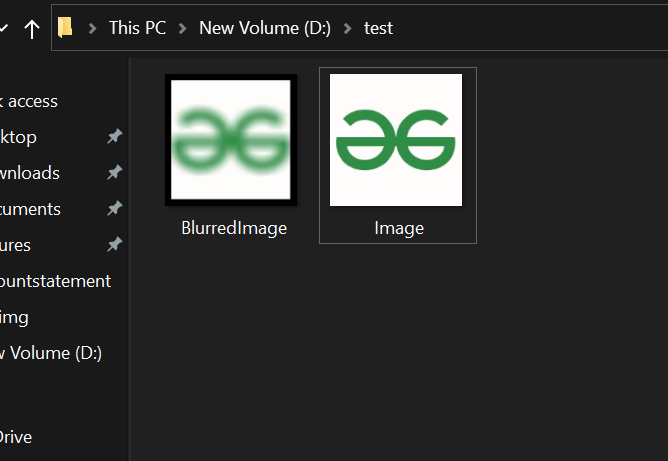GFG App
Open AppBrowser
Continue

# Java Program to Blur Image using Smoothing

Blurring is a simple and frequently used image processing operation. It is also called as Smoothing. Smoothing of an image removes noisy pixels from the image and applying a low-pass filter to an image. A low-pass filter means removing noise from an image while leaving the majority of the image undamaged. The most common type of filter is linear. In a linear filter, the weighted sum of the input pixels value determines the output pixels value.

Prerequisite:

Classes required to perform the operation:

• To read and write an image file we have to import the File class [ import java.io.File; ]. This class represents file and directory path names in general.
• To handle errors we use the IOException class [ import java.io.IOException; ]
• To hold the image we create the BufferedImage object for that we use BufferedImage class [ import java.awt.image.BufferedImage; ]. This object is used to store an image in RAM.
• To perform the image read-write operation we will import the ImageIO class [ import javax.imageio.ImageIO;]. This class has static methods to read and write an image.

Approach:

• Smooth each pixel of image taking as 2D matrix
• Using some in-built methods of BufferedImage class and Color c

Implementation:

Example

## Java

 `// Java Program to Blur Image using Smoothing`   `// Importing required packages` `import` `java.awt.*;` `import` `java.awt.image.BufferedImage;` `import` `java.io.File;` `import` `java.io.IOException;` `import` `javax.imageio.ImageIO;`   `// Main class` `public` `class` `GFG {`   `    ``// Main driver method` `    ``public` `static` `void` `main(String[] args)` `        ``throws` `IOException, InterruptedException` `    ``{`   `        ``Color color[];`   `        ``// Creating a File class object to` `        ``// read the image in the form of file from directory`   `        ``// Directory path is passed as an argument` `        ``File fin = ``new` `File(``"D:/test/Image.jpeg"``);`   `        ``// Now object of BufferedImage class is created to` `        ``// convert file into image form` `        ``BufferedImage input = ImageIO.read(fin);`   `        ``// Again creating an object of BufferedImage to` `        ``// create output Image` `        ``BufferedImage output = ``new` `BufferedImage(` `            ``input.getWidth(), input.getHeight(),` `            ``BufferedImage.TYPE_INT_RGB);`   `        ``// Setting dimensions for the image to be processed` `        ``int` `i = ``0``;` `        ``int` `max = ``400``, rad = ``10``;` `        ``int` `a1 = ``0``, r1 = ``0``, g1 = ``0``, b1 = ``0``;` `        ``color = ``new` `Color[max];`   `        ``// Now this core section of code is responsible for` `        ``// blurring of an image`   `        ``int` `x = ``1``, y = ``1``, x1, y1, ex = ``5``, d = ``0``;`   `        ``// Running nested for loops for each pixel` `        ``// and blurring it` `        ``for` `(x = rad; x < input.getHeight() - rad; x++) {` `            ``for` `(y = rad; y < input.getWidth() - rad; y++) {` `                ``for` `(x1 = x - rad; x1 < x + rad; x1++) {` `                    ``for` `(y1 = y - rad; y1 < y + rad; y1++) {` `                        ``color[i++] = ``new` `Color(` `                            ``input.getRGB(y1, x1));` `                    ``}` `                ``}`   `                ``// Smoothing colors of image` `                ``i = ``0``;` `                ``for` `(d = ``0``; d < max; d++) {` `                    ``a1 = a1 + color[d].getAlpha();` `                ``}`   `                ``a1 = a1 / (max);` `                ``for` `(d = ``0``; d < max; d++) {` `                    ``r1 = r1 + color[d].getRed();` `                ``}`   `                ``r1 = r1 / (max);` `                ``for` `(d = ``0``; d < max; d++) {` `                    ``g1 = g1 + color[d].getGreen();` `                ``}`   `                ``g1 = g1 / (max);` `                ``for` `(d = ``0``; d < max; d++) {` `                    ``b1 = b1 + color[d].getBlue();` `                ``}`   `                ``b1 = b1 / (max);` `                ``int` `sum1 = (a1 << ``24``) + (r1 << ``16``)` `                           ``+ (g1 << ``8``) + b1;` `                ``output.setRGB(y, x, (``int``)(sum1));` `            ``}` `        ``}`   `        ``// Writing the blurred image on the disc where` `        ``// directory is passed as an argument` `        ``ImageIO.write(` `            ``output, ``"jpeg"``,` `            ``new` `File(``"D:/test/BlurredImage.jpeg"``));`   `        ``// Message to be displayed in the console when` `        ``// program is successfully executed` `        ``System.out.println(``"Image blurred successfully !"``);` `    ``}` `}`

Output: On console

`Java Program to Blur Image using Smoothing`

After executing the program console will show a message that Image blurred successfully! This code will not run on an online IDE as it needs an image on disk. It is pictorially depicted in the pictorial format below as follows:My Personal Notes arrow_drop_up